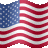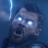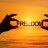# Indicator that find stocks that Pullback to Moving Average

N

#### Nick

##### Member
VIP
Hi, i manage to find this code created by Pete Hahn. It plots the UpArrow these two conditions are met, Price pulls back to 20MA and CCI is below 100. What i did was to apply this indicator and scan together with a uptrending watchlist and the results are satisfactory. You may change the Moving Average by changing the value of ma1.

Code:
``````input length = 14;

def price = close + low + high;

def linDev = lindev(price, length);

def CCI = if linDev == 0 then 0 else (price - Average(price, length)) / linDev / 0.015;

def ma1 = Average(close, 20);

def priceAboveMa1 = close > ma1;

def atLeastFiveBarsAboveMa1 = Lowest(priceAboveMa1, 5) > 0;

def firstTouchInFiveBars = atLeastFiveBarsAboveMa1 and close < ma1;

plot signal = if firstTouchInFiveBars and CCI < 100 then low else Double.NaN;

signal.SetPaintingStrategy(PaintingStrategy.ARROW_UP);

signal.SetDefaultColor(Color.GREEN);``````
Hope this is useful.

Thanks

•Topas, crawmomo and BenTen#### markos

##### Well-known member
VIP
Warehouse
Hi, i manage to find this code created by Pete Hahn. It plots the UpArrow these two conditions are met, Price pulls back to 20MA and CCI is below 100. What i did was to apply this indicator and scan together with a uptrending watchlist and the results are satisfactory. You may change the Moving Average by changing the value of ma1.

Code:
``````input length = 14;
def price = close + low + high;
def linDev = lindev(price, length);
def CCI = if linDev == 0 then 0 else (price - Average(price, length)) / linDev / 0.015;
def ma1 = Average(close, 20);
def priceAboveMa1 = close > ma1;
def atLeastFiveBarsAboveMa1 = Lowest(priceAboveMa1, 5) > 0;
def firstTouchInFiveBars = atLeastFiveBarsAboveMa1 and close < ma1;
plot signal = if firstTouchInFiveBars and CCI < 100 then low else Double.NaN;
signal.SetPaintingStrategy(PaintingStrategy.ARROW_UP);
signal.SetDefaultColor(Color.GREEN);``````
Hope this is useful.
Thanks
Hi @Nick , how does the CCI fit into the puzzle, in your opinion?

N

#### Nick

##### Member
VIP

Hi @markos, i have screenshot two pictures to highlight how the buy arrow will appear. What i did was to use the codes and created a scan query on Daily timeframe. The objective of this scan is to scan for stocks that have met these two conditions, Price touch or close below the 20D MA with the CCI <100 (Oversold) If these two condition is met, an Up Arrow will appear.

Similarly, the CCI can serve to determine when is the Entry Pt, ie BUY is triggered when CCI cuts above the -100 line.

Currently, I am getting some inconsistent scan results as shown in the pics, where, there few arrows are plotted even when the CCI value is not <100.

It will beIf anyone can help to tweak the code, so that we can get the arrows plotted ONLY when the two conditions are present. It will be great

Thanks#### markos

##### Well-known member
VIP
Warehouse
How does the code look in the editor? No errors? The order of operations may be off, just a guess.
Should there be another set of Parenthesis around the CCI line?
Check order of operations in the thinkscript manual, I could be wrong. I don't have ToS available now.
Anyone else?

Last edited:
N

#### Nick

##### Member
VIP
How does the code look in the editor? No errors? The order of operations may be off, just a guess.
Should there be another set of Parenthesis around the CCI line?
Check order of operations in the thinkscript manual, I could be wrong. I don't have ToS available now.
Anyone else?
input tenkan_period = 9;
input kijun_period = 26;
plot Tenkan = (Highest(high, tenkan_period) + Lowest(low, tenkan_period)) / 2;
plot Kijun = (Highest(high, kijun_period) + Lowest(low, kijun_period)) / 2;
plot "Span A" = (Tenkan[kijun_period] + Kijun[kijun_period]) / 2;
plot "Span B" = (Highest(high[kijun_period], 2 * kijun_period) + Lowest(low[kijun_period], 2 * kijun_period)) / 2;
plot Chikou = close[-kijun_period];
Tenkan.SetDefaultColor(GetColor(1));
Kijun.SetDefaultColor(GetColor(2));
"Span A".SetDefaultColor(GetColor(3));
"Span B".SetDefaultColor(GetColor(4));
Chikou.SetDefaultColor(GetColor(5));
DefineGlobalColor("Bullish", Color.YELLOW);
DefineGlobalColor("Bearish", Color.RED);
AddCloud("Span A", "Span B", globalColor("Bullish"), globalColor("Bearish"));
input length = 14;
def price = close + low + high;
def linDev = lindev(price, length);
def CCI = if linDev == 0 then 0 else (price - Average(price, length)) / linDev / 0.015;
def ma1 = Average(close, 10);
def priceAboveCloud = close > "Span A" and close > "Span B";
def priceAboveMa1 = close > ma1;
def atLeastFiveBarsAboveMa1 = Lowest(priceAboveMa1, 5) > 0;
def firstTouchInFiveBars = atLeastFiveBarsAboveMa1 and close < ma1;
plot signal = if firstTouchInFiveBars and CCI < 100 then low else Double.NaN;
signal.SetPaintingStrategy(PaintingStrategy.ARROW_UP);
signal.SetDefaultColor(Color.GREEN);

@markos this is how the original code was written, i realised that i dont really need the ichimoku cloud as another condition to be screened and removed it to just plot uparrow when the price pullbacks to 20MA and CCI is <100.

input length = 14;
def price = close + low + high;
def linDev = lindev(price, length);
def CCI = if linDev == 0 then 0 else (price - Average(price, length)) / linDev / 0.015;
def ma1 = Average(close, 20);

def priceAboveMa1 = close > ma1;
def atLeastFiveBarsAboveMa1 = Lowest(priceAboveMa1, 5) > 0;
def firstTouchInFiveBars = atLeastFiveBarsAboveMa1 and close < ma1;
plot signal = if firstTouchInFiveBars and CCI < 100 then low else Double.NaN;
signal.SetPaintingStrategy(PaintingStrategy.ARROW_UP);
signal.SetDefaultColor(Color.GREEN);

Hope we can tweak the code to achieve the desired results.. thanks#### markos

##### Well-known member
VIP
Warehouse
input tenkan_period = 9;
input kijun_period = 26;
plot Tenkan = (Highest(high, tenkan_period) + Lowest(low, tenkan_period)) / 2;
plot Kijun = (Highest(high, kijun_period) + Lowest(low, kijun_period)) / 2;
plot "Span A" = (Tenkan[kijun_period] + Kijun[kijun_period]) / 2;
plot "Span B" = (Highest(high[kijun_period], 2 * kijun_period) + Lowest(low[kijun_period], 2 * kijun_period)) / 2;
plot Chikou = close[-kijun_period];
Tenkan.SetDefaultColor(GetColor(1));
Kijun.SetDefaultColor(GetColor(2));
"Span A".SetDefaultColor(GetColor(3));
"Span B".SetDefaultColor(GetColor(4));
Chikou.SetDefaultColor(GetColor(5));
DefineGlobalColor("Bullish", Color.YELLOW);
DefineGlobalColor("Bearish", Color.RED);
AddCloud("Span A", "Span B", globalColor("Bullish"), globalColor("Bearish"));
input length = 14;
def price = close + low + high;
def linDev = lindev(price, length);
def CCI = if linDev == 0 then 0 else (price - Average(price, length)) / linDev / 0.015;
def ma1 = Average(close, 10);
def priceAboveCloud = close > "Span A" and close > "Span B";
def priceAboveMa1 = close > ma1;
def atLeastFiveBarsAboveMa1 = Lowest(priceAboveMa1, 5) > 0;
def firstTouchInFiveBars = atLeastFiveBarsAboveMa1 and close < ma1;
plot signal = if firstTouchInFiveBars and CCI < 100 then low else Double.NaN;
signal.SetPaintingStrategy(PaintingStrategy.ARROW_UP);
signal.SetDefaultColor(Color.GREEN);

@markos this is how the original code was written, i realised that i dont really need the ichimoku cloud as another condition to be screened and removed it to just plot uparrow when the price pullbacks to 20MA and CCI is <100.

input length = 14;
def price = close + low + high;
def linDev = lindev(price, length);
def CCI = if linDev == 0 then 0 else (price - Average(price, length)) / linDev / 0.015;
def ma1 = Average(close, 20);

def priceAboveMa1 = close > ma1;
def atLeastFiveBarsAboveMa1 = Lowest(priceAboveMa1, 5) > 0;
def firstTouchInFiveBars = atLeastFiveBarsAboveMa1 and close < ma1;
plot signal = if firstTouchInFiveBars and CCI < 100 then low else Double.NaN;
signal.SetPaintingStrategy(PaintingStrategy.ARROW_UP);
signal.SetDefaultColor(Color.GREEN);

Hope we can tweak the code to achieve the desired results.. thanks
@Nick Try to leave everything in the code, it will not take up memory or be recognized if you just comment out the lines you don't want.
So, just put a # in front of each line you want out and it will be ignored.
That way you will see if any of the code you want is somehow dependent on the code you don't want.
Otherwise, try to change the ICHI stuff to (Color.Current) and hopefully that will make the color transparent. (I hope, it's just a guess)
Next, go through the Universe and the ToS manuals.
Another "thunk", CCI is a built in study, try to just reference that vs the linDev lines as they might point to the ICHIstuff...

•Nick
N

#### Nick

##### Member
VIP
@Nick Try to leave everything in the code, it will not take up memory or be recognized if you just comment out the lines you don't want.
So, just put a # in front of each line you want out and it will be ignored.
That way you will see if any of the code you want is somehow dependent on the code you don't want.
Otherwise, try to change the ICHI stuff to (Color.Current) and hopefully that will make the color transparent. (I hope, it's just a guess)
Next, go through the Universe and the ToS manuals.
Another "thunk", CCI is a built in study, try to just reference that vs the linDev lines as they might point to the ICHIstuff...
@markos thank you

•markosQuestions 5Questions 10Questions 2